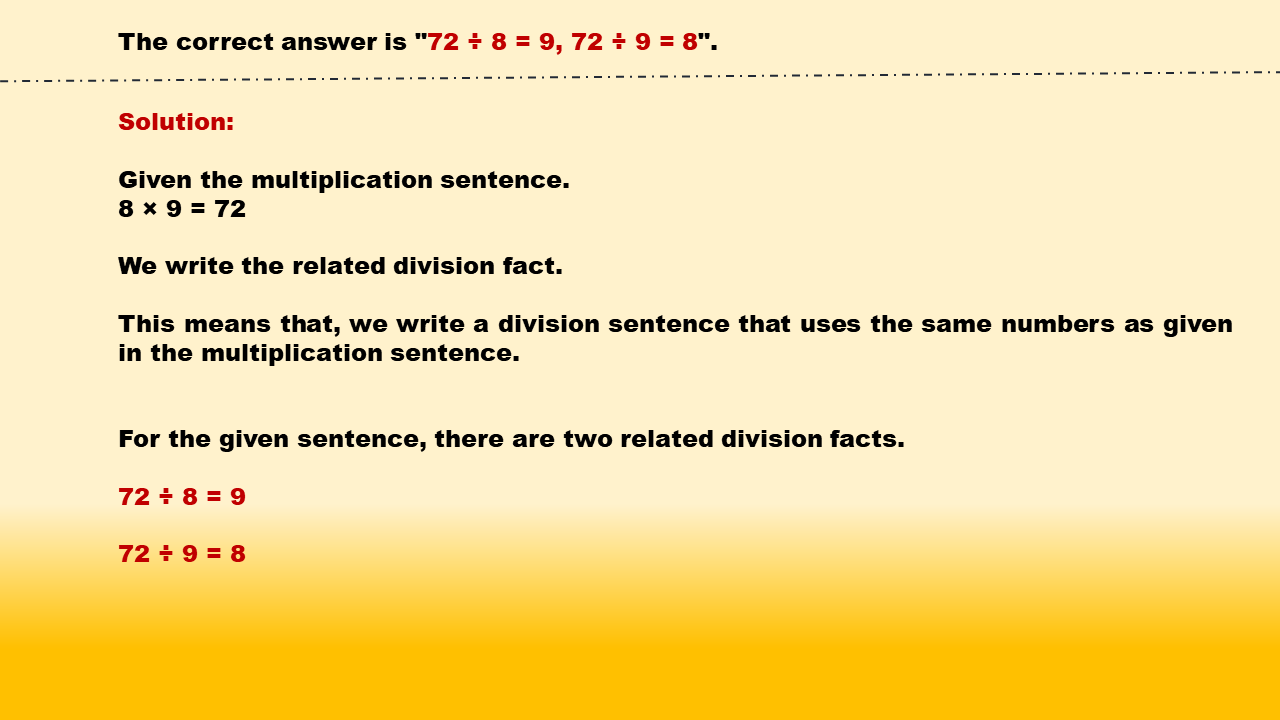1
visibility

Identify related division facts for 8 × 9 = 72.

• 45 ÷ 9 = 5, 45 ÷ 5 = 9

• 72 ÷ 9 = 8, 72 ÷ 8 = 9

• 30 ÷ 6 = 5, 30 ÷ 5 = 6

• 24 ÷ 8 = 3, 24 ÷ 3 = 8